# Decimals on a Number Line

Decimals on a Number Line• To read decimals on a number line, look at how many parts there are between each whole number.
• If there are 10 parts, the decimals count up in tenths, increasing by 0.1 each time.
• If there are 5 parts, the decimals count up in fifths, increasing by 0.2 each time.
• If there are 4 parts, the decimals count up in quarters, increasing by 0.25 each time.
• If there are 2 parts, the decimals count up in halves, increasing by 0.5 each time.

The number of parts between each whole number tells us what the decimals are increasing by each time.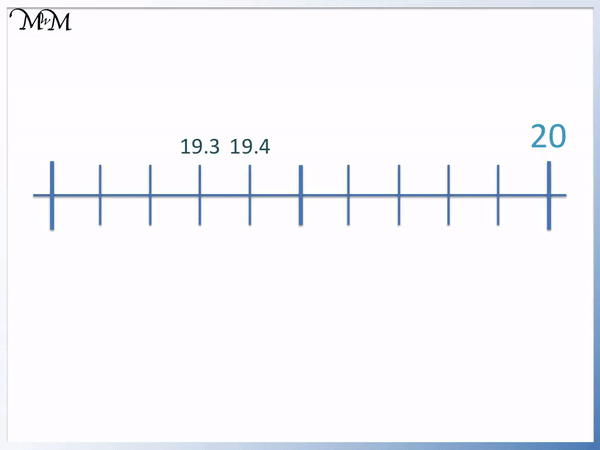• There are ten equal parts between 19 and 20 and so each increment is one tenth.
• Moving from one line to the next increases the decimal by 0.1.
• We can count backwards from 19.3 to get to 19.2, 19.1 and then 19.
• We can count up from 19.4 to get to 19.5, 19.6, 19.7, 19.8 and 19.9.
• The two decimal numbers marked by the arrows are 19.2 and 19.8#### Decimals on a Number Line Video – Hundredths# Decimals on a Number Line

## How to Represent Decimals on a Number Line

Decimals on a number line are written depending on the number of parts between each whole number.

Number of parts Amount to increase by
100 0.01
10 0.1
5 0.2
4 0.25
3 0.33
2 0.5First count the number of parts in between each whole number.

Then use the table above to decide what each increment increases by.

Count up by this amount as you go from one line to the next.

For example, this number line contains 5 parts between each whole number. This means that the increments increase by 0.2 each time.The digit after the decimal point will increase by 2 each time.

The marked decimals on the number line are 25.4 and 26.8.

## Negative Decimals on a Number Line

To represent negative decimals on a number line, first mark the negative numbers on the line to the left of zero. Divide each whole number up into the required number of parts. The negative decimals are a mirror image of the positive decimal numbers with the numbers decreasing as we move left from zero.

Here is an example of plotting the negative decimals on a number line between -0.1 and -0.2.Between -0.1 and -0.2 is one tenth. We have divided this tenth into ten further parts and therefore the decimals go up and down in hundredths.

We have -0.11, -0.12 and so on as we move left.

Here is another example of plotting negative decimals.

We have the negative decimals shown between -2.5 to -2.7. Each tenth is divided into five parts and so we go up and down by 0.02.We go from -2.5 to -2.52 to -2.54 etc. until we reach -2.6. We then have -2.62, -2.64 until we reach -2.7.## Fifths on a Number Line

Whenever there are 5 equal parts between each whole number on a number line, we count up in fifths. Each fifth is worth 0.2. To count up in fifths on a number line, increase the digit after the decimal point by 2 each time.

One fifth is double the size of one tenth.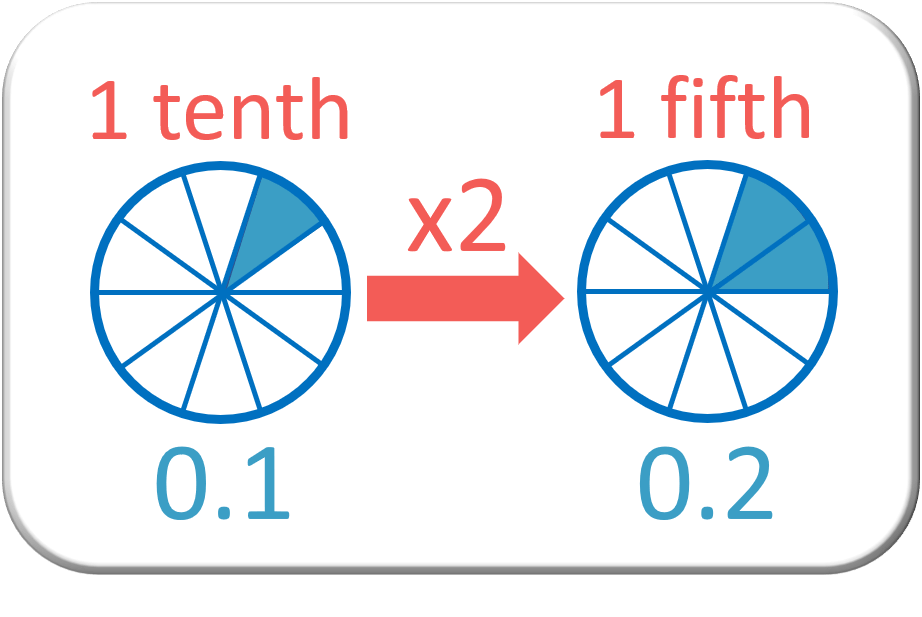One fifth is 2 tenths so it is worth 0.2.We can see that 5 fifths make a whole. Adding 5 lots of 0.2 makes 1.For example, find the missing decimals marked on the number line below.

Because each whole number is divided into 5 parts, we know we have fifths, increasing by 0.2 each time.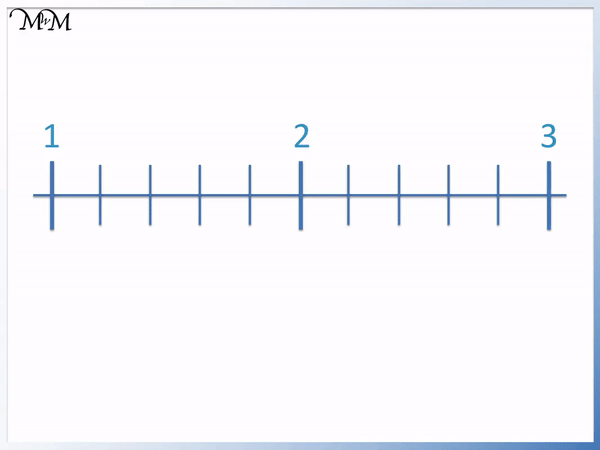Counting on in 0.2s from 1, we have the first marked decimal as 1.6 and the second marked decimal as 2.4.

## Tenths on a Number Line

Tenths are written on a number line by counting up by 0.1. Tenths are shown whenever there are ten equal parts between each whole number. To count up in tenths on a number line, increase the digit after the decimal point by 1 each time.

For example, if there are 10 parts separating 14 and 15 on a number line then we count up in tenths. The lines represent 14.1, 14.2, 14.3 etc.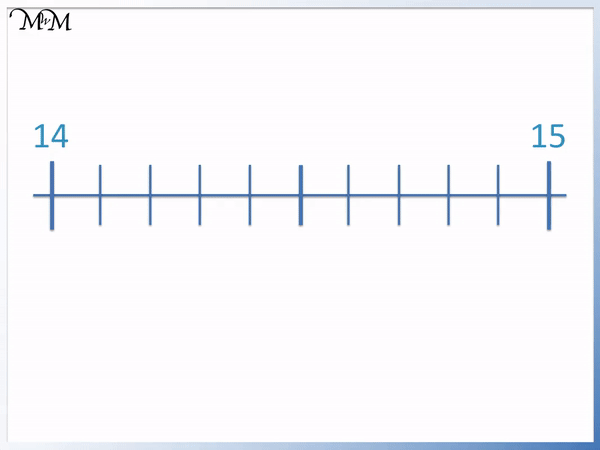Once we reach 14.9, the next tenth up is just 15.

## Hundredths on a Number Line

Hundredths are shown on a number line whenever there are 100 parts between each whole number or if there are 10 equal parts between each tenth We count up by 0.01 when counting up in hundredths.

For example, here there are ten parts separating 0.1 and 0.2, so we count up in hundredths.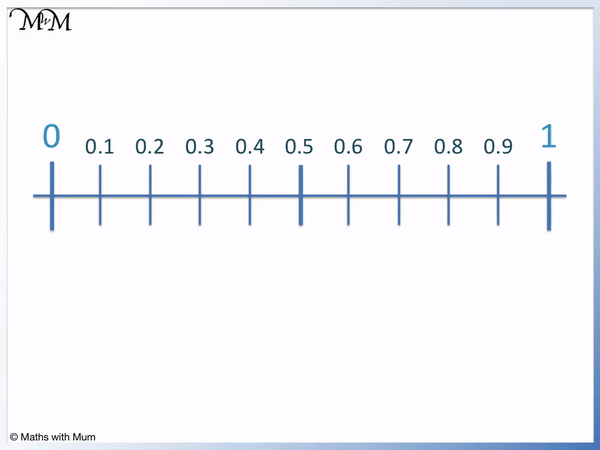We can think of 0.1 as 0.10 and 0.2 as 0.20. This allows us to see that between 0.10 and 0.20 we have 0.11, 0.12, 0.13 and so on.Since the tenths were divided into ten more parts, we now formed hundredths. Each decimal increases by 0.01.

Here is another example of representing hundredths on a number line.

We have the tenths of 1.1, 1.2 and 1.3 marked on the number line. Each tenth is divided into ten further parts and so, we have hundredths.

Each decimal increases by 0.01 each time.## Teaching Decimals on a Number Line

When teaching decimals on the number line, first identify any key numbers on the number line, such as whole numbers or halves. Count the number of parts in between each key number so we can identify how much to increase by each time.

Use the following chart to decide how much we increase by each time.Now try our lesson on Rotational Symmetry where we learn how to find the order of rotational symmetry for a shape.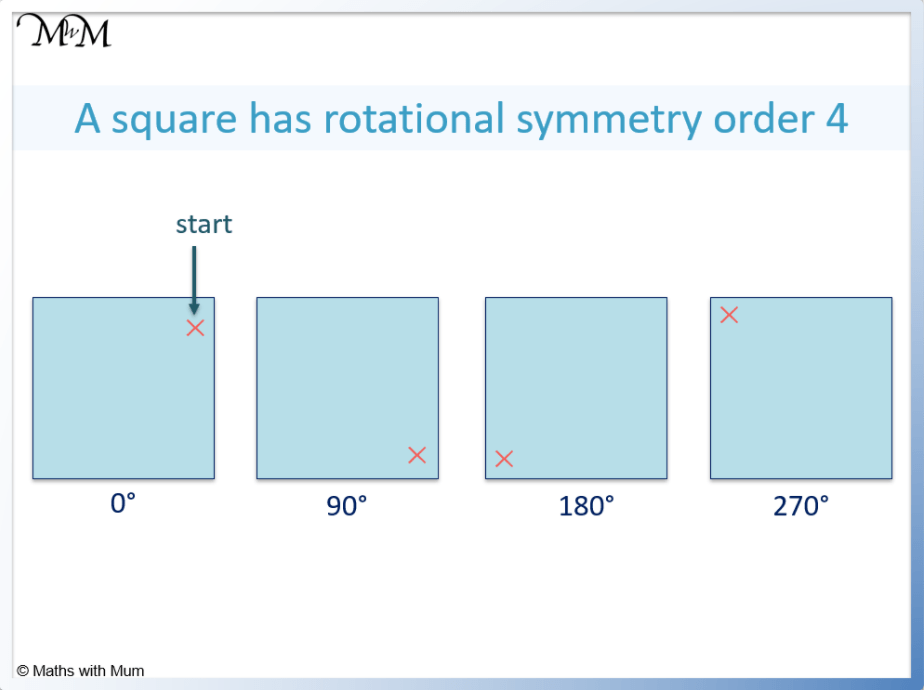error: Content is protected !!